Courses
Courses for Kids
Free study material
Free LIVE classes
More

# 3 Digit Addition without CarryingLIVE
Join Vedantu’s FREE Mastercalss

## Introduction to Addition of 3 Digit Numbers

Three-digit addition is the process of adding two or more three-digit numbers. The two numbers' total is the name given to the outcome. There are two techniques to add three digits: addition with regrouping, sometimes known as the carrying forward approach, and addition without regrouping.

An added and an augend are combined to find a total. The addend is sometimes referred to as an addend. The term total can also be referred to as the sum. We will discuss 3-digit addition without carrying today.

There are four basic operations of arithmetic, i.e., addition, subtraction, multiplication, and division. Today, we will discuss addition or, to be more precise, three-digit addition without carrying. The addition is the process of adding two or more numbers together, and the result obtained is known as the sum of the given numbers. Every arithmetic operation has its unique symbol. In addition, the symbol is ‘+’.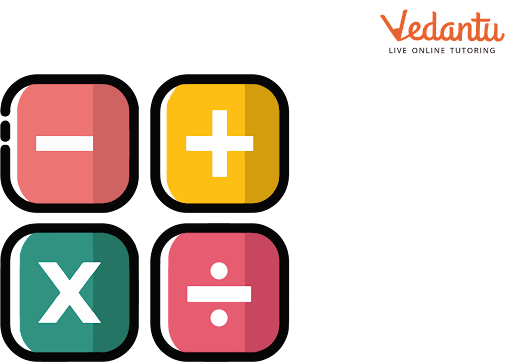Arithmetic Operations

In addition to 3 digits, the place value of the numbers is given more importance. The place value is expressed here as ones, tens, and hundreds. To add 3 digits numbers, arrange them column-wise according to their place values. Digits are added one by one. The numbers added are called addends, and the answer obtained is known as their sum.

3 digit addition can be done in two ways. First is the ‘regrouping’ method, also known as carrying forward, and the ‘without regrouping’ method, also known as no carrying forward.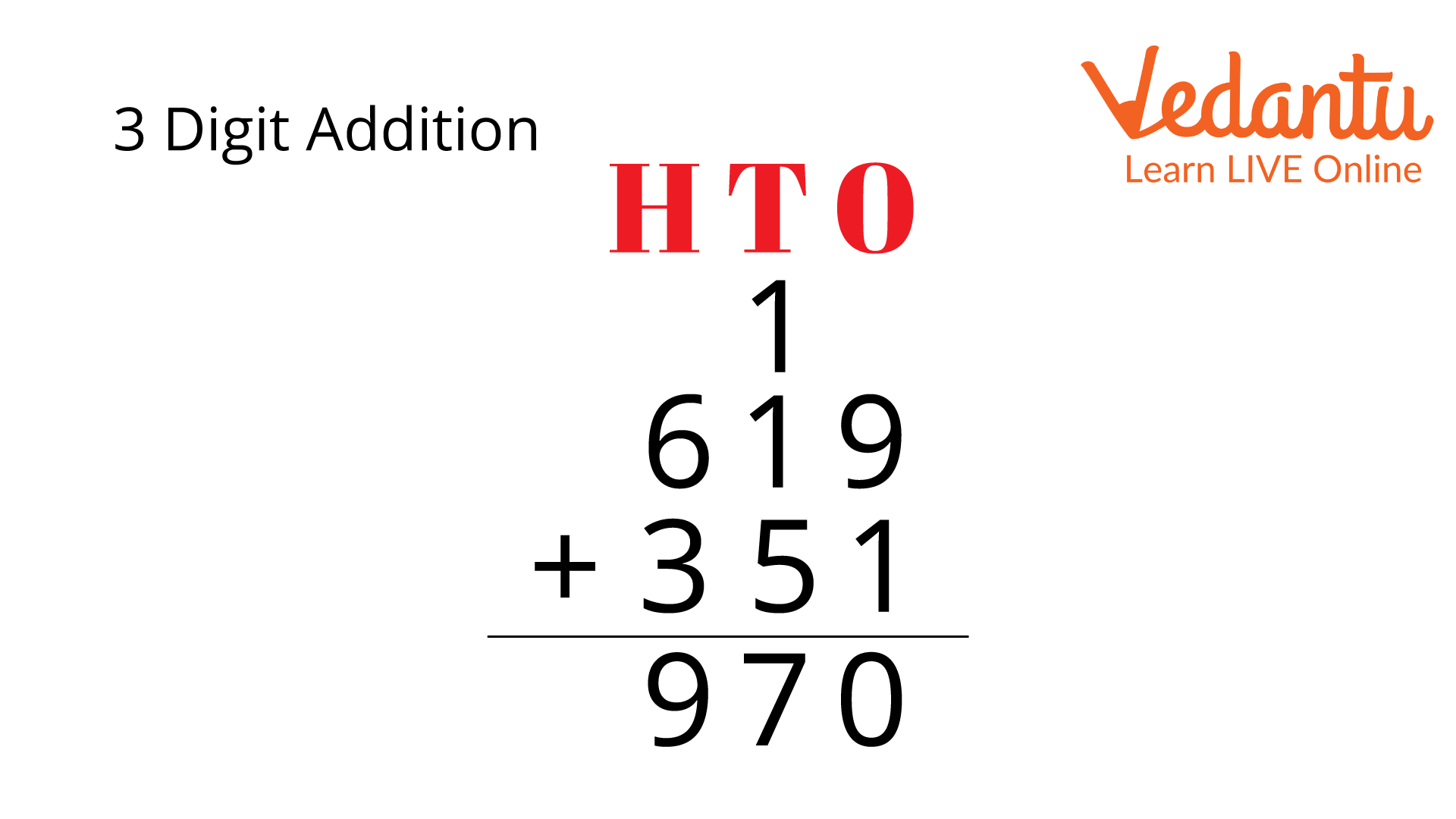3 Digits Addition with Carry Forward Method

### 1. Addition of 3 Digit Numbers with Regrouping:

There is a condition in the ‘regrouping’ method, also known as the carrying forward method. Here, we have to move the extra number to the next column if the sum of the numbers in either of the columns is more than 9. We begin from the one's column and move left towards the tens and hundreds of columns.

Let us take an addition of 3 digits of the addition of 528 and 298.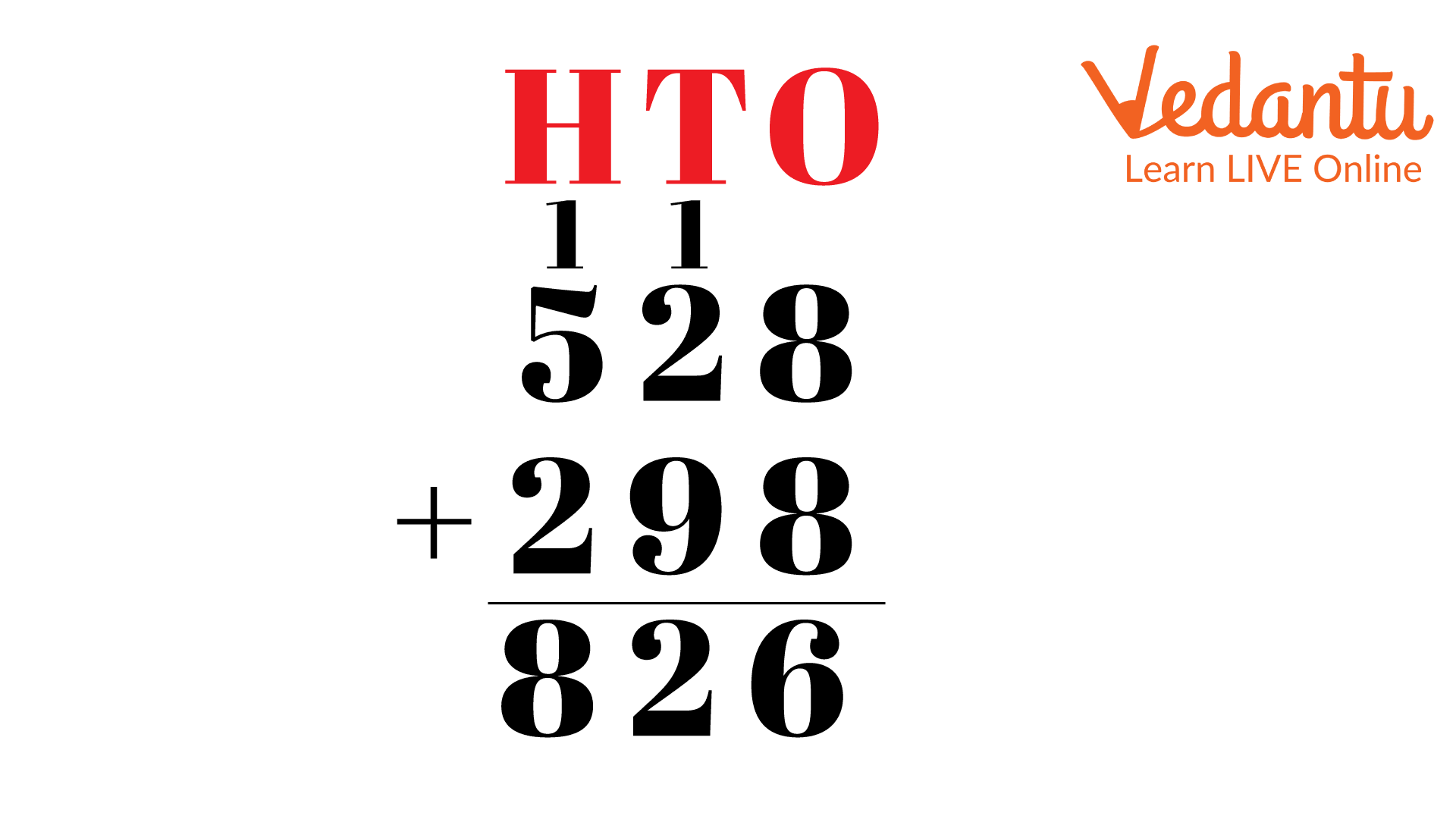$528 + 298$

If we start adding from one’s column, we get $8{\rm{ }} + {\rm{ }}8{\rm{ }} = {\rm{ }}16$, with 1 as the carrying number.

The tens column already has a carrying number, so $2{\rm{ }} + {\rm{ }}9{\rm{ }} + {\rm{ }}1{\rm{ }} = {\rm{ }}12$ with 1 as the carrying number.

The hundreds column already has a carrying number, so we get $5{\rm{ }} + {\rm{ }}2{\rm{ }} + {\rm{ }}1{\rm{ }} = {\rm{ }}8$.

Therefore, the result is 826.

This example can be declared as 3 digit number addition with regrouping.

### 2. Addition of 3 Digit Numbers without Regrouping:

The ‘Without regrouping’ method is very simple, also known as the carrying forward method. It is used in our day-to-day lifestyle, like shopping and any transaction. Here, when the sum is less than or equal to 9, we write the number according to its place value column, which does not carry forward any number.

Let us take an addition of 3 digits of the addition of 558 and 231.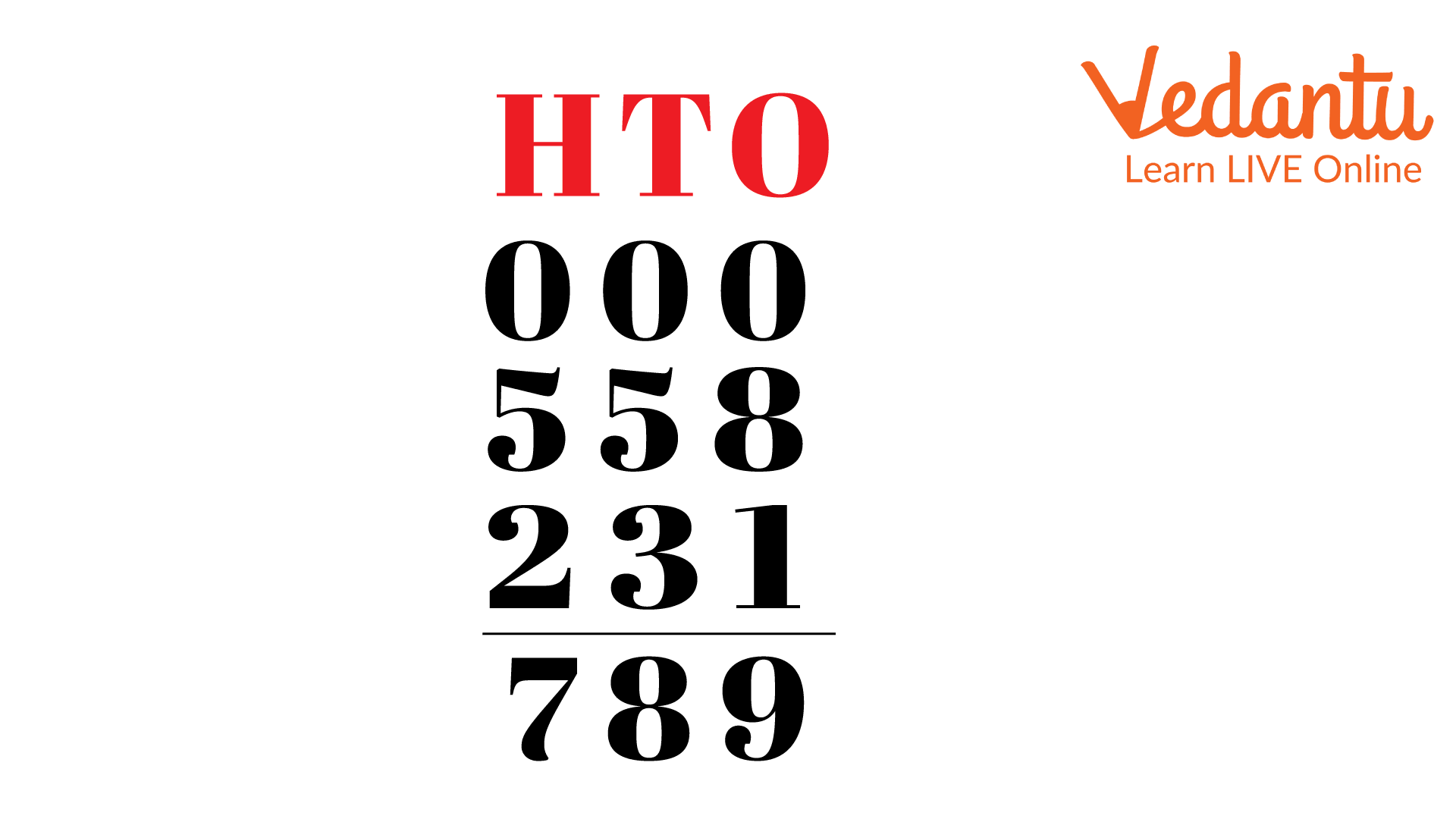$558 + 231$

If we start adding from one’s column, we get $8{\rm{ }} + {\rm{ }}1{\rm{ }} = {\rm{ }}9$, and no extra carry number.

For the tens column, we get $5{\rm{ }} + {\rm{ }}3{\rm{ }} = {\rm{ }}8$ and no extra carry number.

For the hundreds column, we get $5{\rm{ }} + {\rm{ }}2{\rm{ }} = {\rm{ }}7$ with no extra carry number.

Therefore, the result is 789.

This example can be declared as 3-digit addition numbers without regrouping.

Now, let us solve some 3-digit addition with carry-over examples or 3 Digit Addition with carry-over worksheets given below:

Example 1:

Add the 3-digit numbers: 232 and 172.

Solution:

First, we will have to add the digits of the one's column, then the tens and then the hundreds. So, let us get started.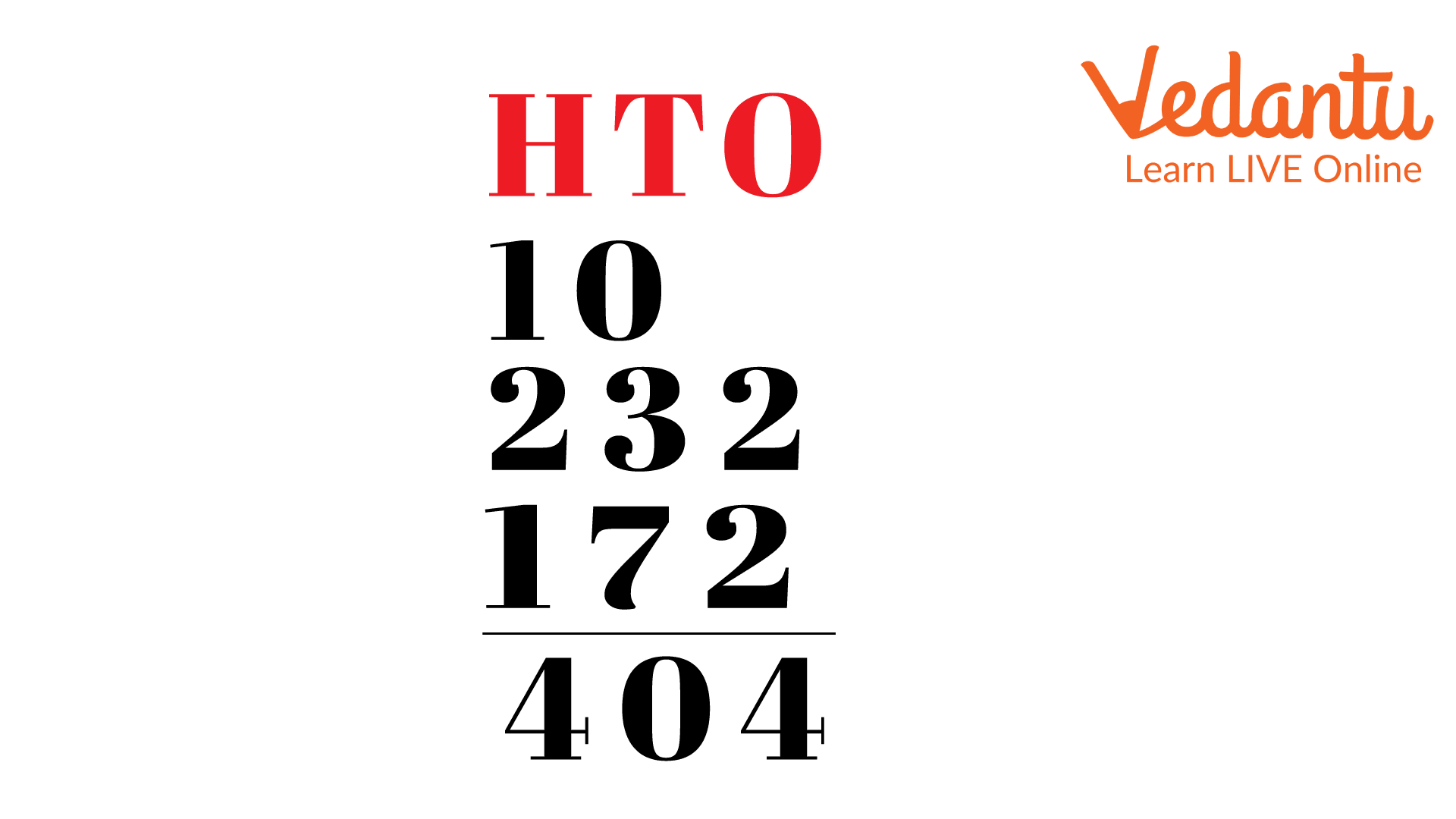$232 + 172$

Numbers in the ones columns: $2{\rm{ }} + {\rm{ }}2{\rm{ }} = {\rm{ }}4$.

As the sum of the one's columns is less than 9, we do not have a carry-over number.

Numbers in the tens columns: $3{\rm{ }} + {\rm{ }}7{\rm{ }} = {\rm{ }}10$.

As the sum of the tens columns is more than 9, we have a carry-over number of 1.

Numbers in the hundreds columns along with the carry over number: $2{\rm{ }} + {\rm{ }}1{\rm{ }} + {\rm{ }}1{\rm{ }} = {\rm{ }}4$.

Therefore, the result we obtained is $232{\rm{ }} + {\rm{ }}172{\rm{ }} = {\rm{ }}404$.

Example 2:

Add the 3-digit numbers: 511 and 109.

Solution:

First, we will have to add the digits of the one's column, then the tens and then the hundreds. So, let us get started.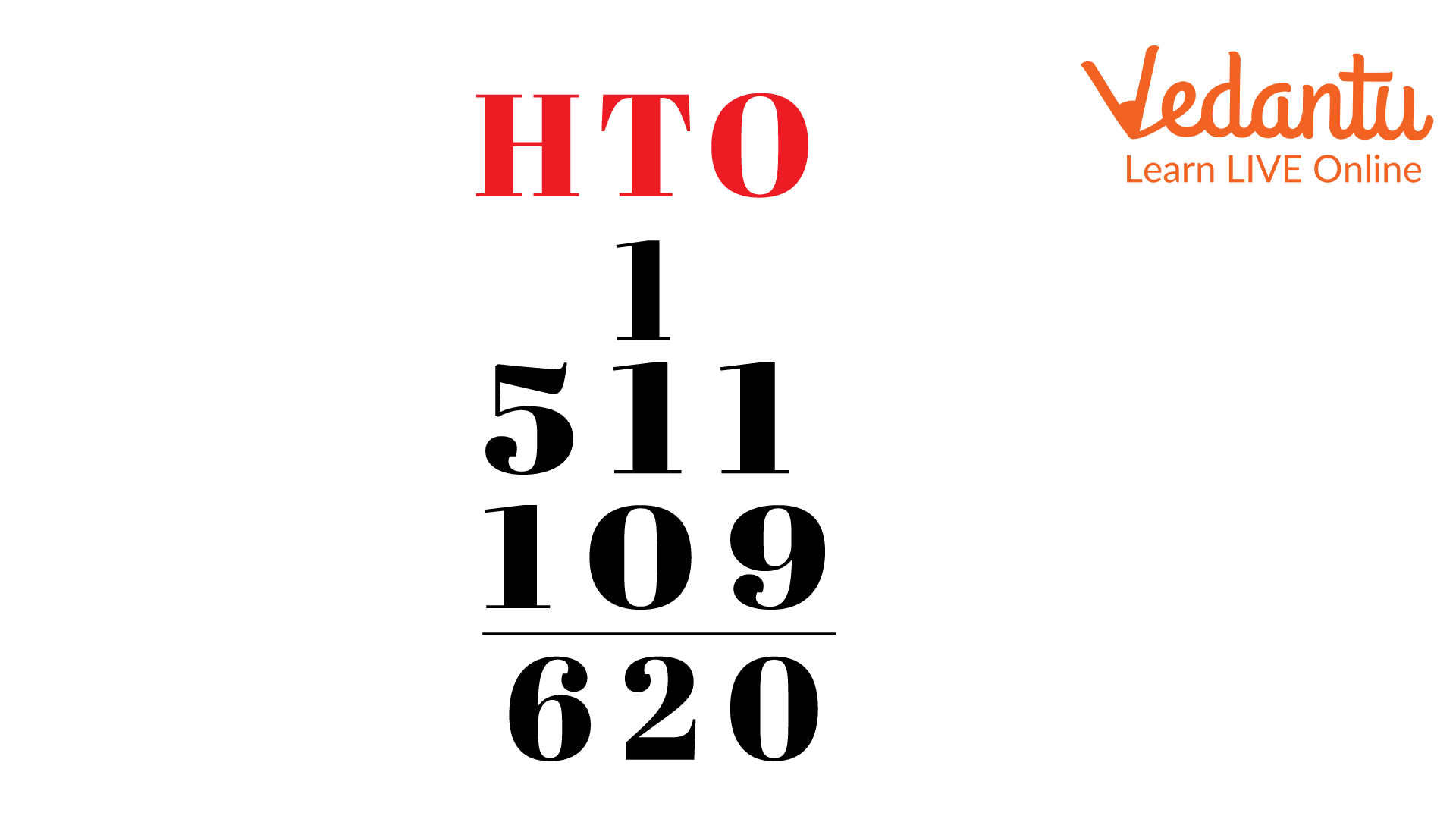$511 + 109$

Numbers in the ones columns: $1{\rm{ }} + {\rm{ }}9{\rm{ }} = {\rm{ }}10$.

As the sum of the one’s columns is more than 9, we have a carry-over number of 1.

Numbers in the tens columns along with the carry over number: $1{\rm{ }} + {\rm{ }}0{\rm{ }} + {\rm{ }}1{\rm{ }} = {\rm{ }}2$.

As the sum of the one's columns is less than 9, we do not have a carry-over number.

Numbers in the hundreds columns: $5{\rm{ }} + {\rm{ }}1{\rm{ }} = {\rm{ }}6$.

Therefore, the result we obtained is $511{\rm{ }} + {\rm{ }}109{\rm{ }} = {\rm{ }}620$.

Example 3:

Perform addition of $228{\rm{ }} + {\rm{ }}228$.

Solution:

First, we will have to add the digits of the one's column, then the tens and then the hundreds. So, let us get started.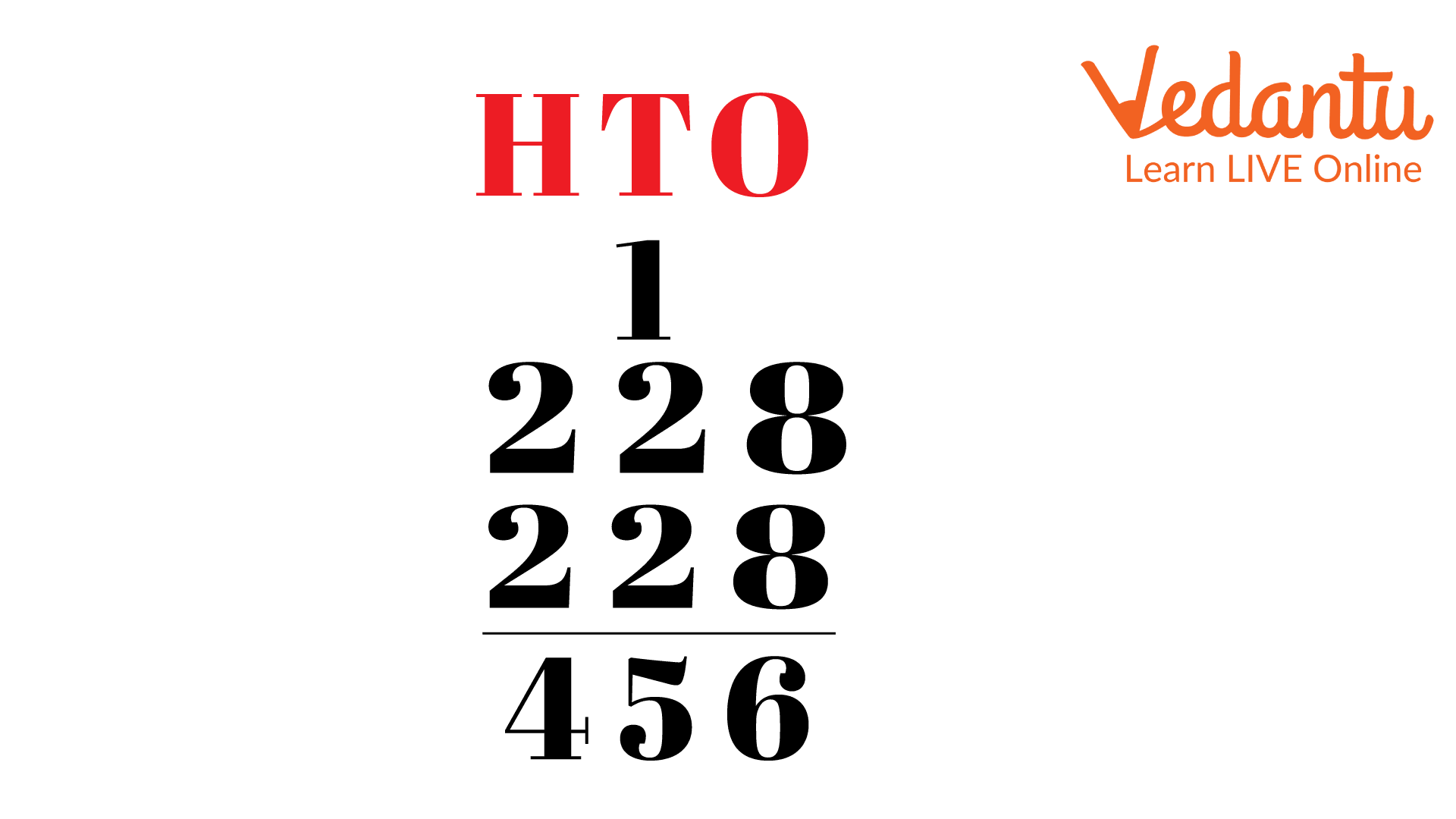$228 + 228$

Numbers in the ones columns: $8{\rm{ }} + {\rm{ }}8{\rm{ }} = {\rm{ }}16$.

As the sum of the one’s columns is more than 9, we have a carry-over number of 1.

Numbers in the tens columns along with the carry-over number: $2{\rm{ }} + {\rm{ }}2{\rm{ }} + {\rm{ }}1{\rm{ }} = {\rm{ }}5$.

As the sum of the one's columns is less than 9, we do not have a carry-over number.

Numbers in the hundreds columns: $2{\rm{ }} + {\rm{ }}2{\rm{ }} = {\rm{ }}4$.

Therefore, the result we obtained is $228{\rm{ }} + {\rm{ }}228{\rm{ }} = {\rm{ }}456$.

## Conclusion

The addition is one of the four basic operations of Mathematics, with the other three being subtraction, multiplication, and division. The addition means finding the total or sum by combining two or more numbers. If the addition of the digits is more than 9 in each column, it is termed as addition with regrouping. And If the addition of the digits is less than 9 in each column, it is termed as an addition without regrouping.

Last updated date: 22nd Sep 2023
Total views: 92.1k
Views today: 1.92k

## FAQs on 3 Digit Addition without Carrying

1. How is 3-digit addition taught to a child?

It can be done using the digital open number line method. Take an example for better understanding; if a child is adding 225 and 443, the student will start at one of the numbers on the number line: 225. Then, add the four hundred: 400, making it 625. Next, add the tens: 40, making it 485. Finally, adding the ones: 3, coming to their answer, 483.

2. What is the main rule of addition?

The result of adding two positive numbers will also have a positive sign. When a positive number and a negative number are added, the operation and the result are determined by the sign of the larger number.

3. What is the principle of addition?

According to the addition principle, an equation is still true if the same integer is added to both sides. If some value is added to one side, it must be added to the other side of the equation. It is also known as the addition fact.